# 0.展示PTA总分

#### 一维数组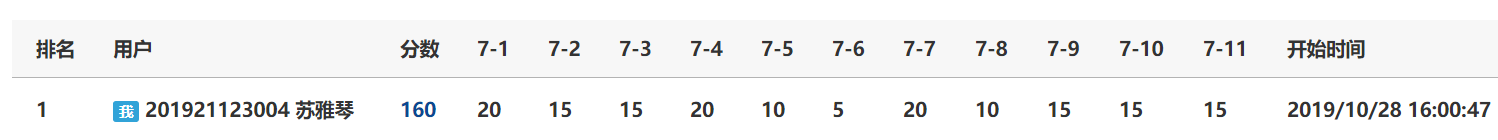#### 二维数组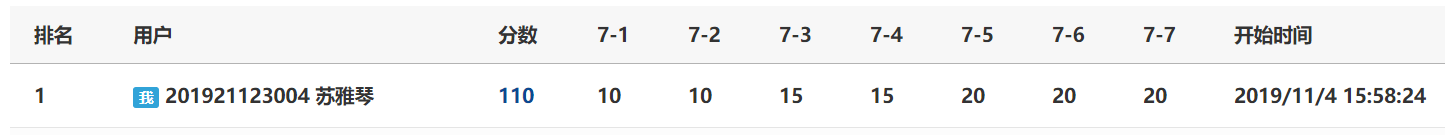#### 字符数组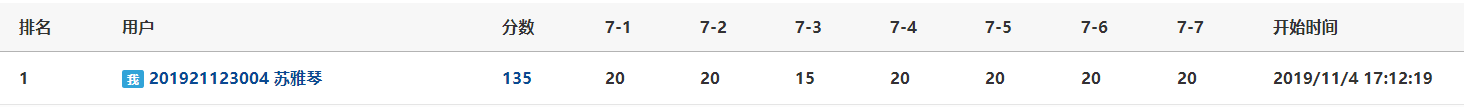# 1.本章学习总结

## 1.1学习内容总结

### 一维数组知识点：

#### 1：一维数组的输入和遍历：

• 输入：
for(i=0;i<n;i++)
{
scanf("%d",&a[i]);
}
遍历
for(i=0;i<n;i++)
{
printf("%d",&a[i]);
}



#### 2:一维数组查找数据：

• 顺序查找法：将数存入数组，再输入一个数x，在数组中一个一个查找，看是否有相同的数存在,存在即输出相应的下标。

• for(i=0;i<n;i++)
{
if(a[i]==x)
{
printf("index is %d",i);//输出相应下标

}

• 查找最小值及其所对应的下标

• /*找最小值a[index] */
index=0;  /*假设最小值是a,即下标为0的元素最小
for(i=1;i<n;i++)
{
if(a[i]<a[index])//如果a[i]比假设的值还小
{
index=i;//再假设a[i]是新的最小值，即下标为i的元素最小
}
}


#### 3.一维数组：在有序数列（从小到大排列）中插入数据

• 伪代码

• 定义数组数n，插入数x;循环变量i，j；
定义数组a[n]，插入数的位置loc;
for i=0 to i<n
输入数对数组赋值
end for
输入要插入的数x
for j=0 tp n
如果x小于a[j]xunc
则把下标存为loc；
然后立刻退出循环
end for
for i=N to i>loc //因为要多插入一个数，所以数组有n+1个数
a[i]=a[i-1]; //从后面开始对数进行存放
end for
让下标为loc的数组位置存放插入数x
for i=0 to i=N
对数组进行遍历；
end for


简单案例：

7-3 简化的插入排序

#### 4.一维数组：删除数据

• **方法一：重构数组 **

• 在原数组上对数组进行删减

•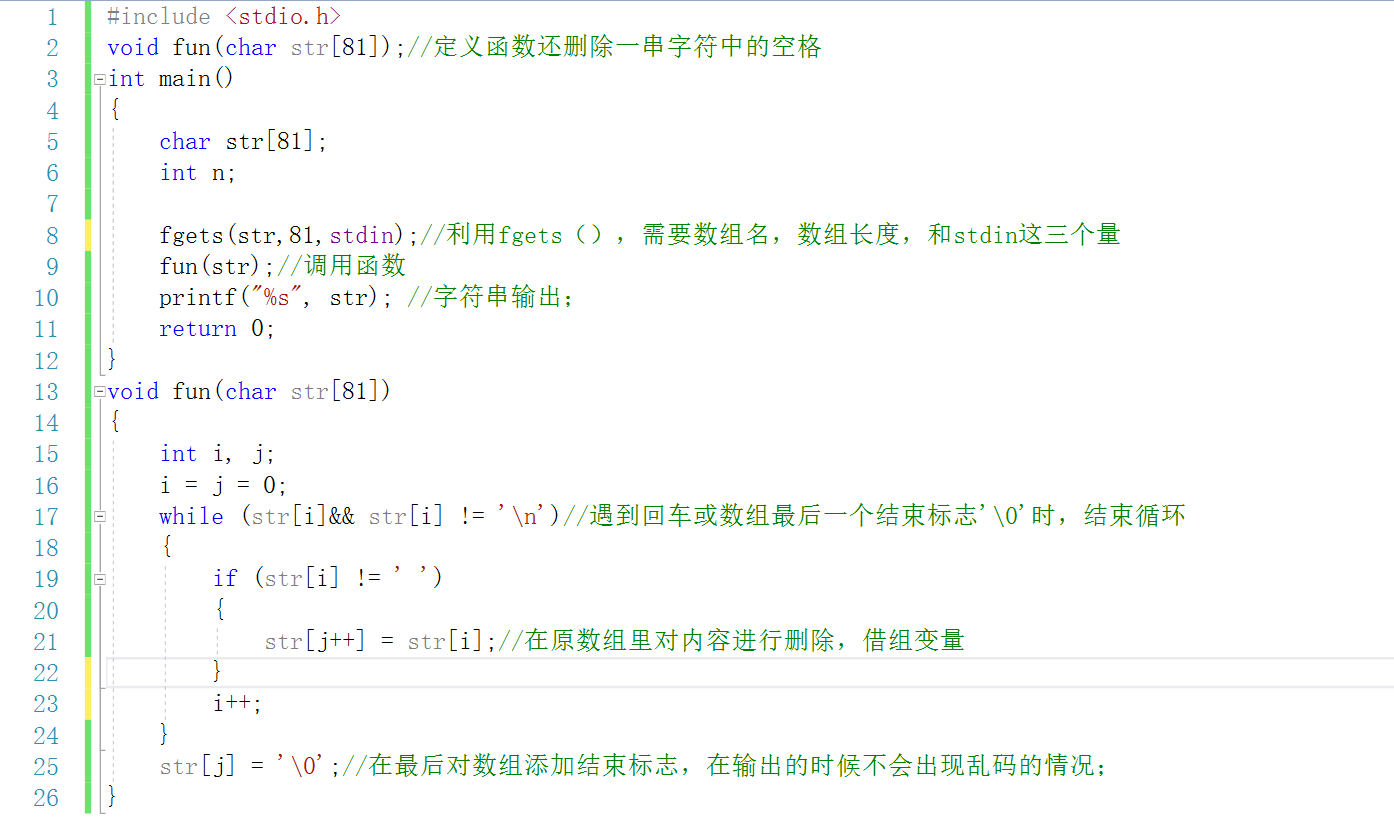• 在原数组上都数据进行删减是不太适合的做法，我们一般保留原数组，再增加一个数组，对其进行赋值，然后遍历。

• 代码如下：

•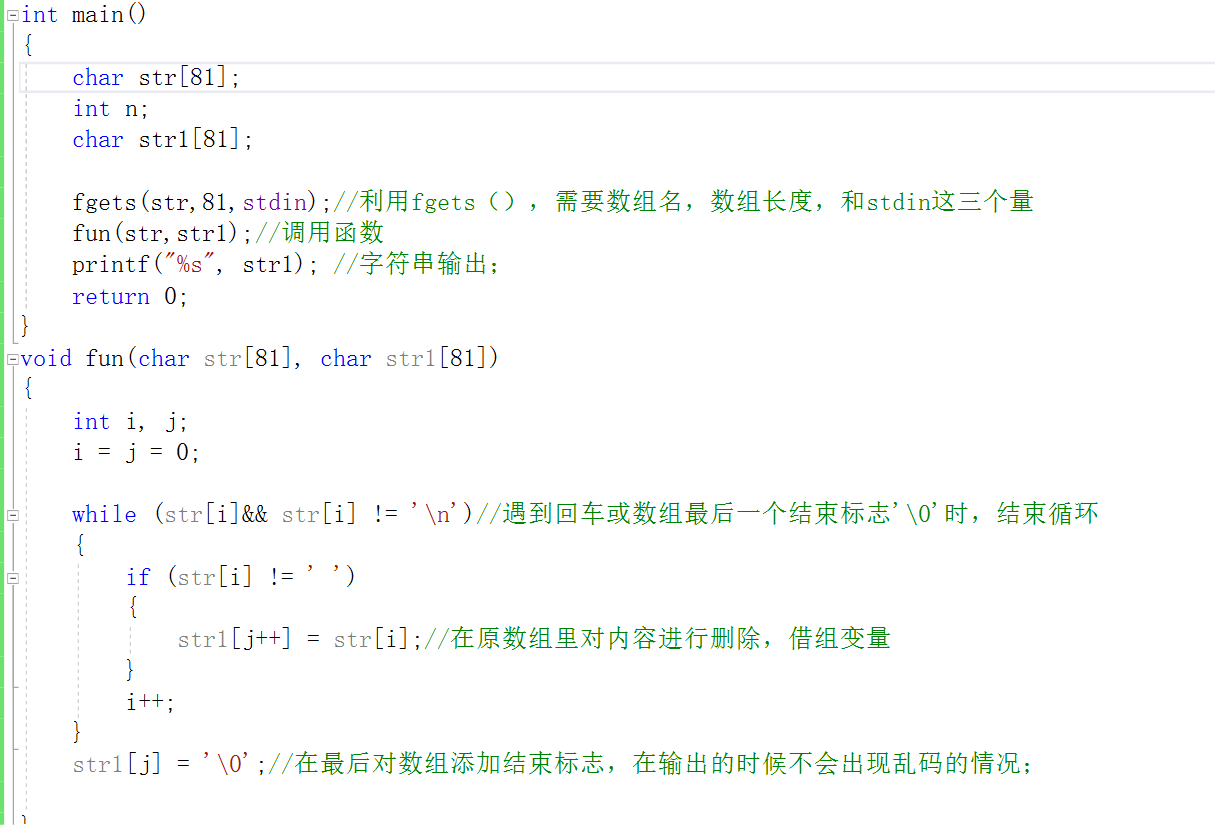• 方法二：与在数组中插入数相似

• 代码如下：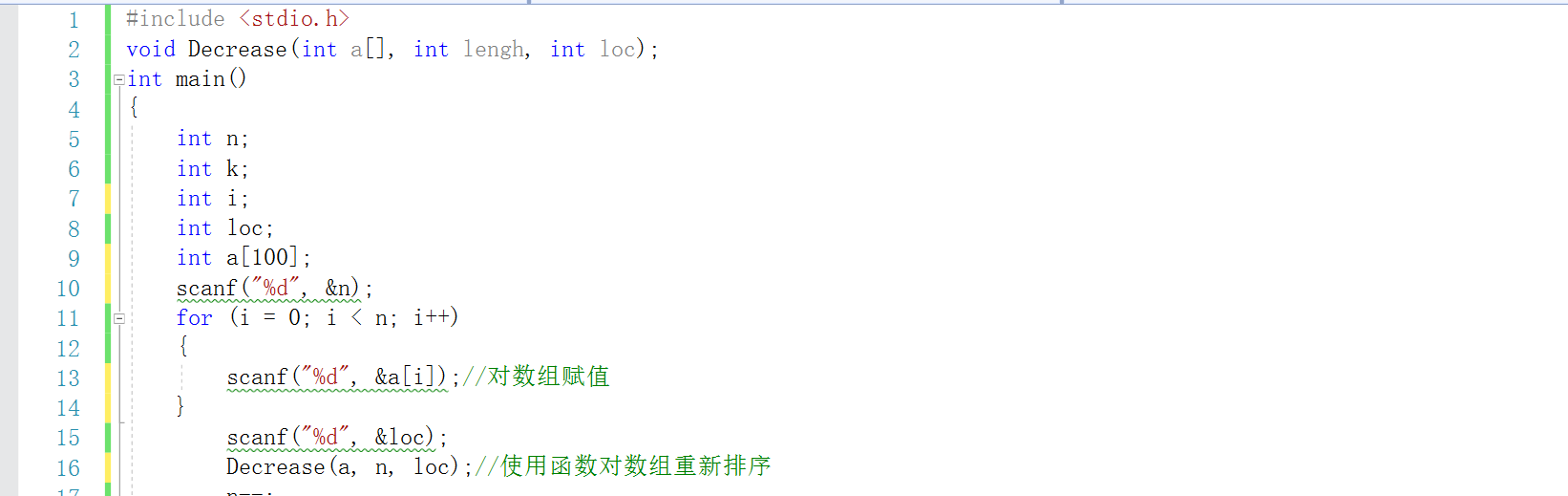•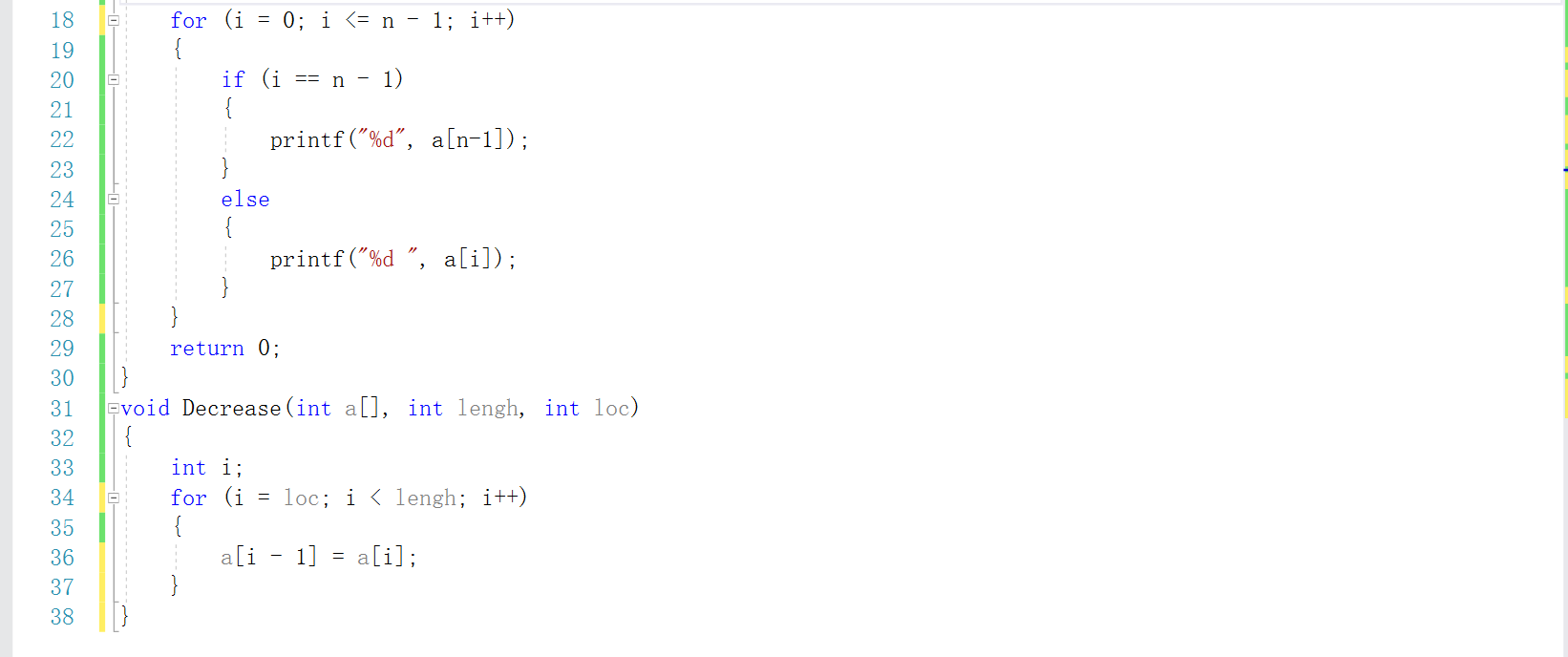• 简单案例：

7-6 数组元素的删除 (5 分)

#### 5.一维数组：排序方法

• a：选择排序法

• 算法步骤：
第一步：在未排序的n个数（a~a[n-1])中找到最小数，将它与a交换；
第二步：在剩下的未排序的n-1个数（a~a[n-1])中找到最小数，将它与a交换；
......
第n-1步，在剩下的未排序的2个数（a[n-2]~a[n-1])中找到最小数，将它与a[n-2交换；

• 伪代码

定义数组的大小n，循环遍历i，j；
定义大小为n的数组a[n];
中间变量term，存放最小值下标index；
for i=0 to i<n
将数存进数组中；
end for
for i=0 to i<n-1 //对数进行n-1趟的比较
index=i;  //设最小下标是第一个数
for j=0 to j<=n-1 //寻找最小值所在下标
if(a[index]>a[j])
index=i;end if
end for
然后使用中间变量对数a[index]和a[i]进行互换
end for
最后对数组进行遍历

• 输入样例：3 5 2 8 1

• 变化过程如下：

• J index a a a a a 说明
3 5 2 8 1
0 4 1 5 2 8 3 a~a中最小值是a,a与a对换
1 2 1 2 5 8 3 a~a中最小值是a,a与a对换
2 4 1 2 3 8 5 a~a中最小值是a,a与a对换
3 4 1 2 3 5 8 a~a中最小值是a,a与a对换
• 简单案例：

实验7-1-5-一维数组 选择法排序

• b：，冒泡排序法

• 算法步骤：
在第一次循环时。把a和a比较，由于它们不符合次序要求，对它们要做交换，然后把a和a比较。以此类推。第一次循环的效果时把数组最大元素“冒泡”到a[n-1]。第二次循环后，不再检查a[n-2].即不再改变它，第二次循环，则把次大数存放在a[n-2]中，以此类推。

• 伪代码：

• 定义变量数组数n。循环变量；
中间变量t。数组a[n];
for i=1 to i<n //外部循环
for j=0 to j<n-i //内部循环
if(a[j]>a[j+1])//相邻两个数比较
{
t=a[j];a[j]=a[j+1];a[j+1]=t;/如果前一个数大，则交换
}
end for
end for

• 输入样例：7 3 66 3 -5 22 -77 2

• 7 3 66 3 -5 22 -77 2
第一遍 3 7 3 -5 22 -77 2 66
第二遍 3 3 -5 7 -77 2 22 66
第三遍 3 -5 3 -77 2 7 22 66
第四遍 -5 3 -77 2 3 7 22 66
第五遍 -5 -77 2 3 3 7 22 66
第六编 -77 -5 2 3 3 7 22 66
第七遍 -77 -5 2 3 3 7 22 66
• 代码如下：

•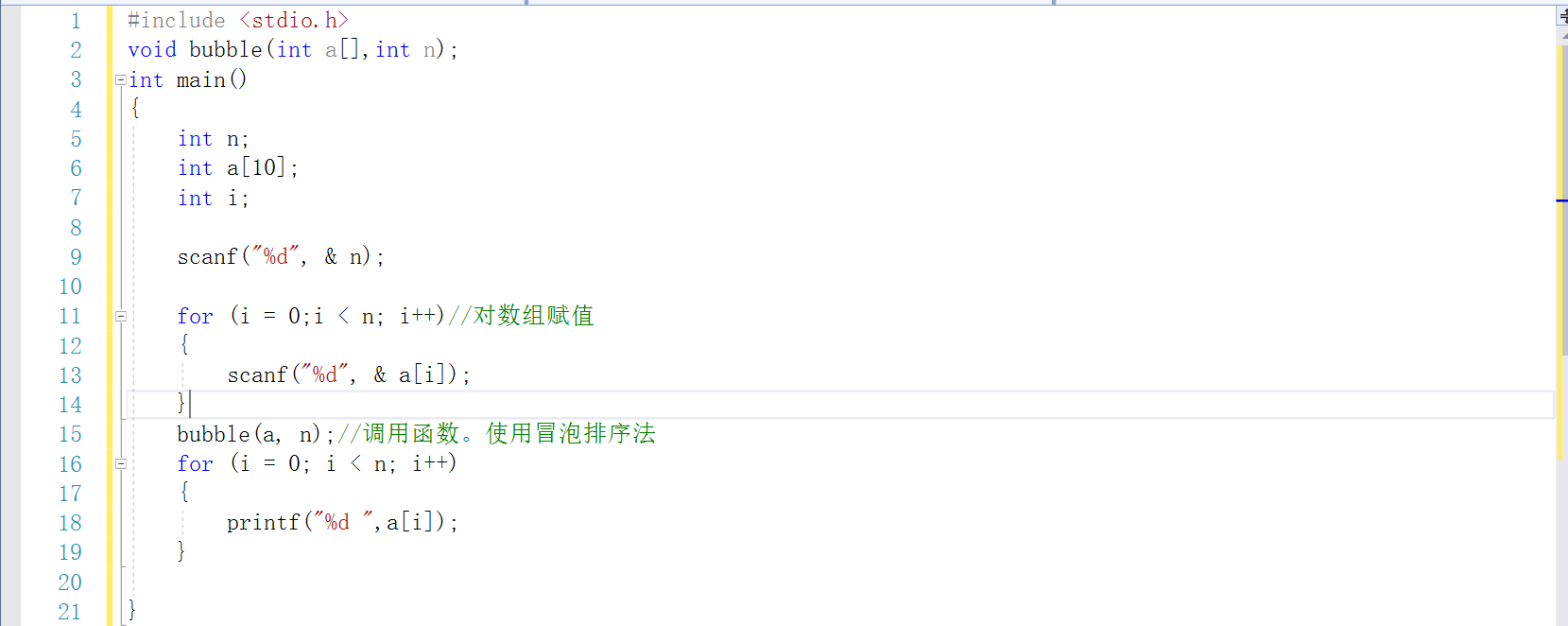•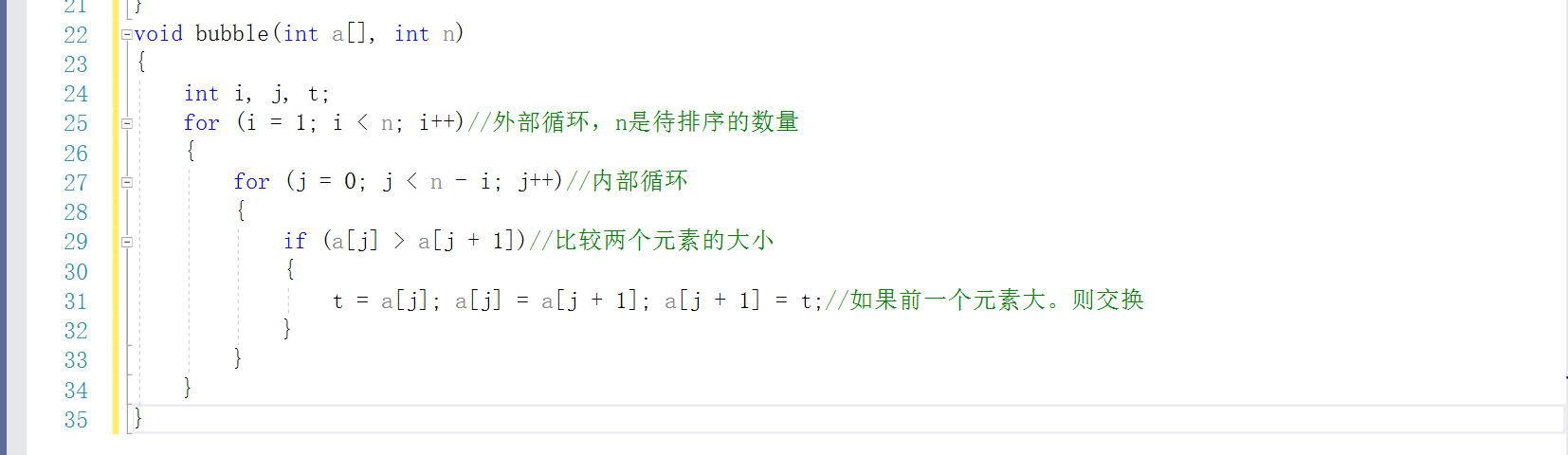#### 6.数组做枚举

• 十进制转n进制

• 代码如下：

•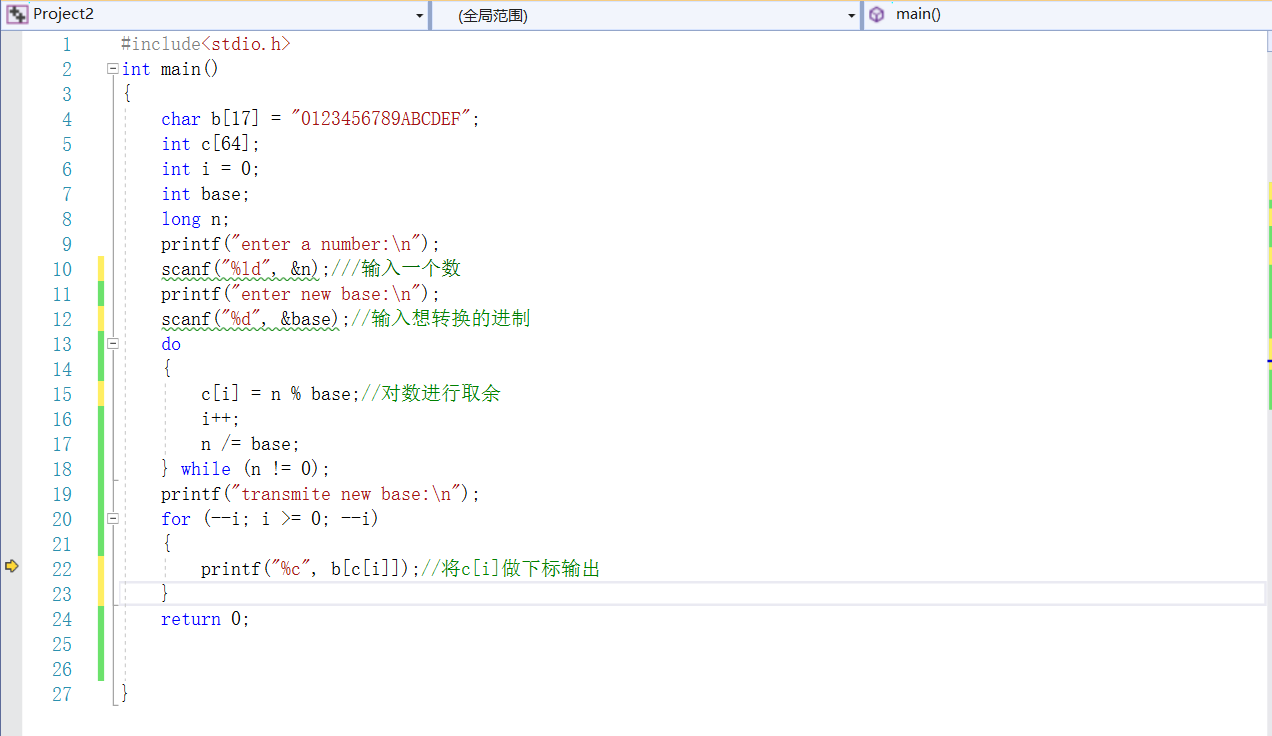• 小贴士：b数组存储各种进制的数，枚举了16进制中各个数的值。

#### 7.一维数组：哈希数组用法

• 判断重复数据

• 代码如下：

•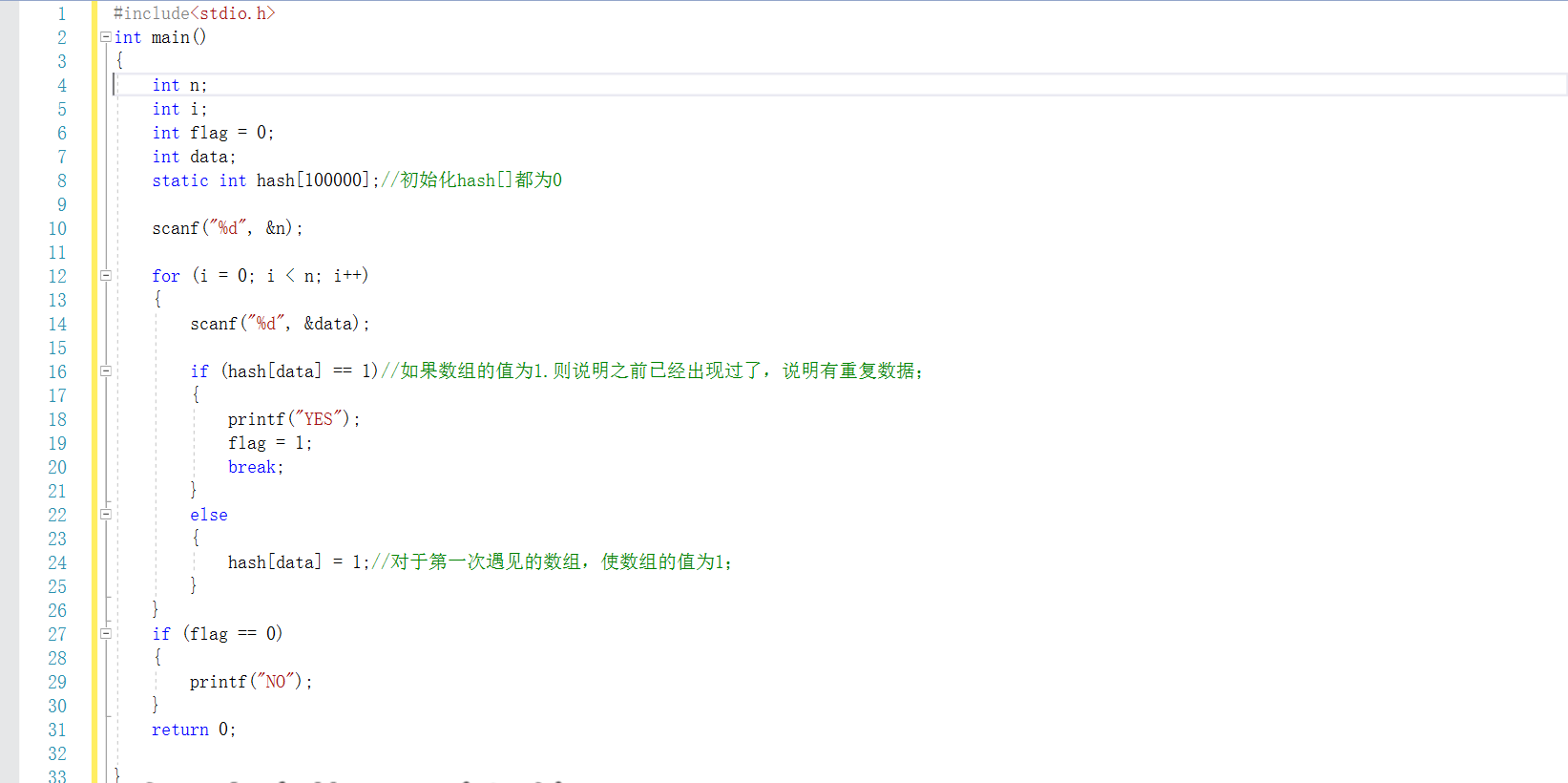• 小贴士：使用这个方法，会比传统的对数组进行一次又一次的遍历比较来的更高更快。输入的数据可以使正整数和字符型数据，但是如果是小数点和负数则不可以用这个方法。

##### 8.数组的左移和右移
• 代码如下：

•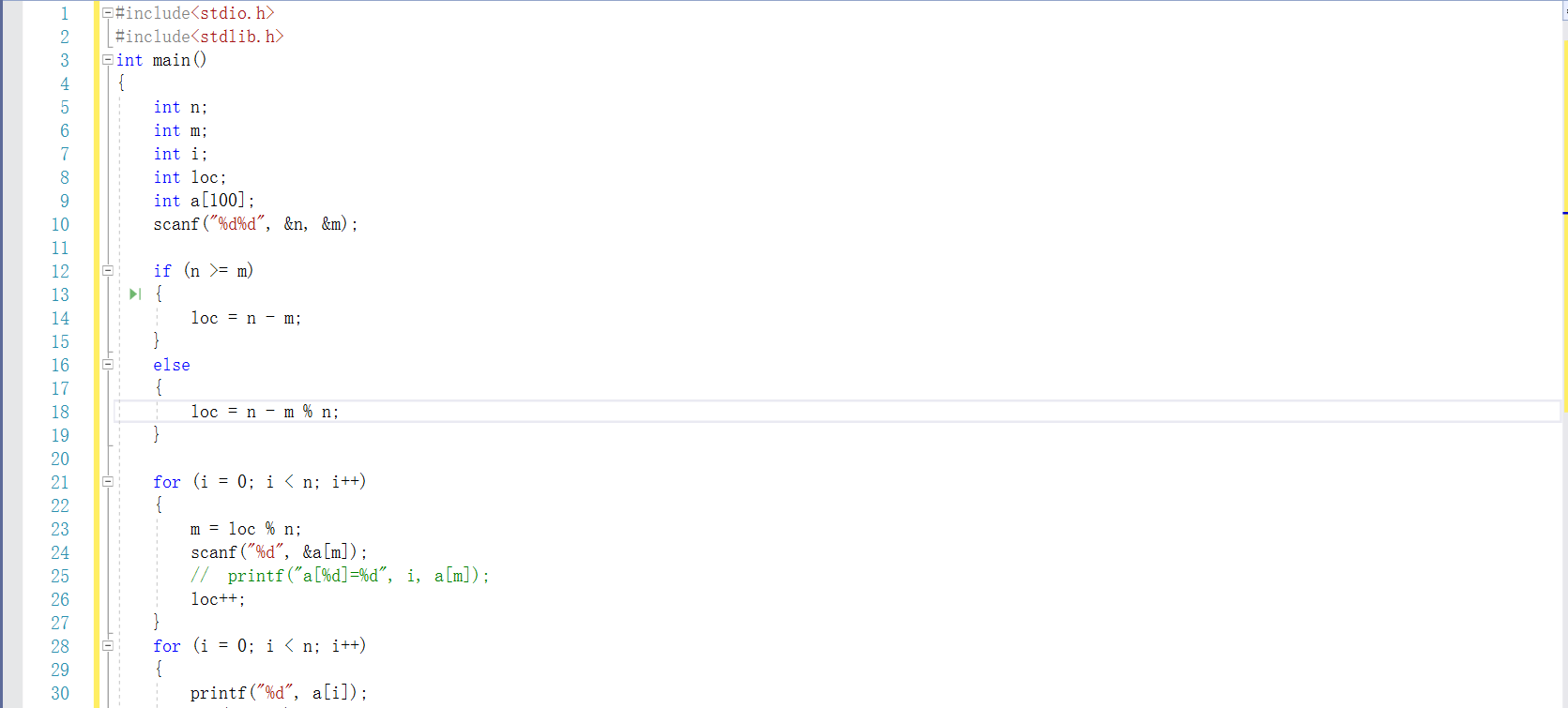•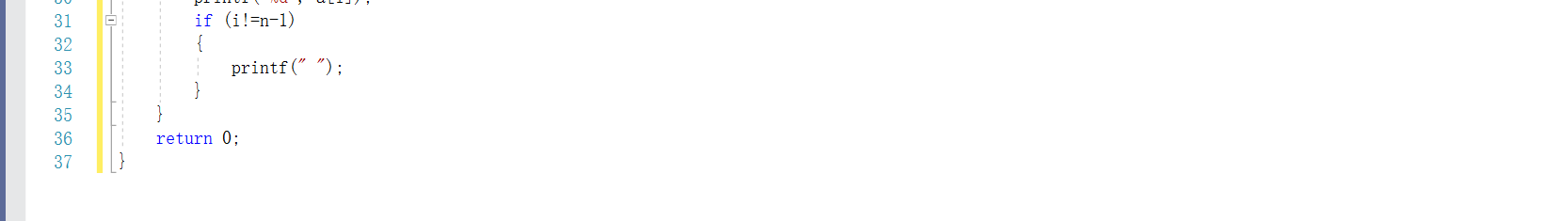• 简单案例：
实验7-2-7-二维数组 方阵循环右移

### 二维数组：

#### 8.二维数组的输入和遍历

输入：
for(i=0;i<n;i++)
{
for(j=0;j<n;j++)
scanf("%d",&a[i][j]);
}

for(i=0;i<n;i++)
{
for(j=0;j<n;j++)
printf("%d",&a[i][j]);
}


#### 9.对二维数组的列或行进行从小到大的排列

• 伪代码

• 定义数组a[n][m].循环i.j,k;
最小值下标index；中间变量term
for i=0 to i<n
for j=0 to j<m
对数组进行赋值；
end for;
for j=0 to j<m
for i=0 to i<n-1
index=i;
for k=i+1 to k<n
如果a[index][j]大于a[k][j]
那么index=k
end for
交换a[index][j]和a[i][j]的值
end for


• 简单案例：

7-1 二维数组每列排序

• 小贴士：对于二维数组的排序，本质上还是与一维数组排序的一样，不过多了一层循环。

### 一维字符数组：

• 一维字符数组的定义，引用，初始化与其它类型的一维数组一样，不同的是，在定义一个字符数组时，c会为字符串自动加入结束标识'\0',所以我们在定义字符串数组的时候。要注意字符串的存储容量。

• 一维数组的初始化：

• static char s[]={"happy"};
static char s[]="happy";

• 字符串遇'\0'结束，第一个'\0'前面的所有字符和'\0'一起构成字符串。所以在对字符数组进行赋值时，在循环外应该对字符数组再赋'\0'值.

#### 10.对字符数组使用循环时的语句

##### a：
while((str[i]=getchar())!='\n')
i++;
str[i]='\0';//输入结束符

##### b:
for(i=0;str[i]||str[i]!='\0';i++)
{
putchar(syr[i]);
}

##### 11.关于读取字符串的函数
• fgets（数组名，数组数，stdin）；

• fgets()函数读取到换行符。文件尾，或读完n-1个字符时结束，包括换行符。如果输入的字符长度小于n-1，会多带一个换行符。

• 循环条件这样设置：

• for（i=0;str[i]&&str[i]!='\n';i++)


#### 12.字符进制转换

• 如果是数字字符，则是n=n*base+ch[i]-'0';
• 如果是字母字符，则是n=n*base+ch[i]-'a'+10;或n=n *base +ch[i]-'A'+10

1500

# 2.PTA实验作业

## 2.1 螺旋方阵

题目：所谓“螺旋方阵”，是指对任意给定的N，将1到N×N的数字从左上角第1个格子开始，按顺时针螺旋方向顺序填入N×N的方阵里。本题要求构造这样的螺旋方阵。


### 2.1.1伪代码

定义两个数组，b={1}用来判断数组a已经有值的地方，就不用再对有值的地方输入东西。

for i=0 to i<n
for j=0 to j<n

end for
whbiaoshile(当最中间的数不等于n的平方)
{
while (y < n && b[x][y] == 0)//y<n把值赋到第一行最后一个数，然后b[][]遇到外围是1时停止
{
a[x][y] = t;
b[x][y] = 1;//对a[i][j]赋过值的地方让b[i][j]为1
t++; y++; count++;
}
y--；x++；
下面同理
}



## 2.1.2代码截图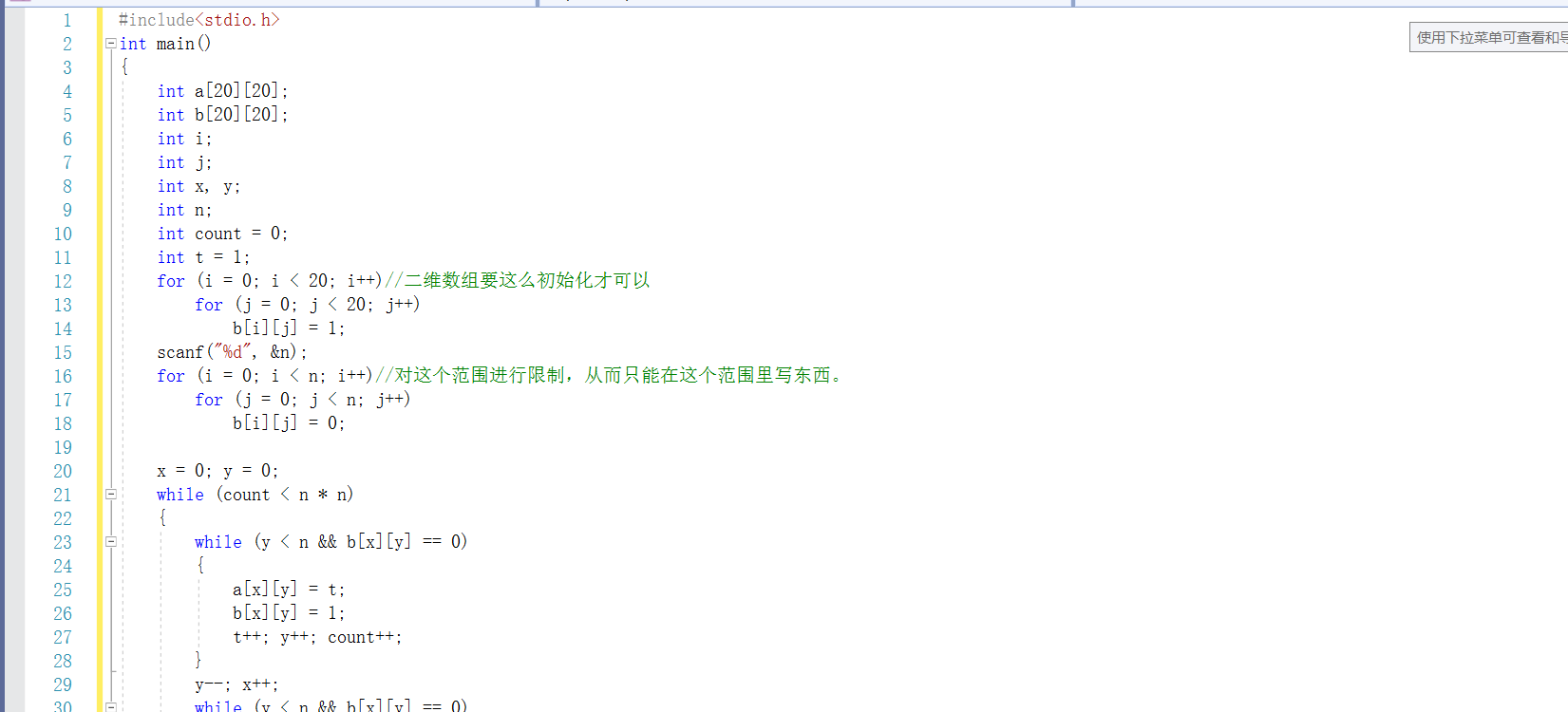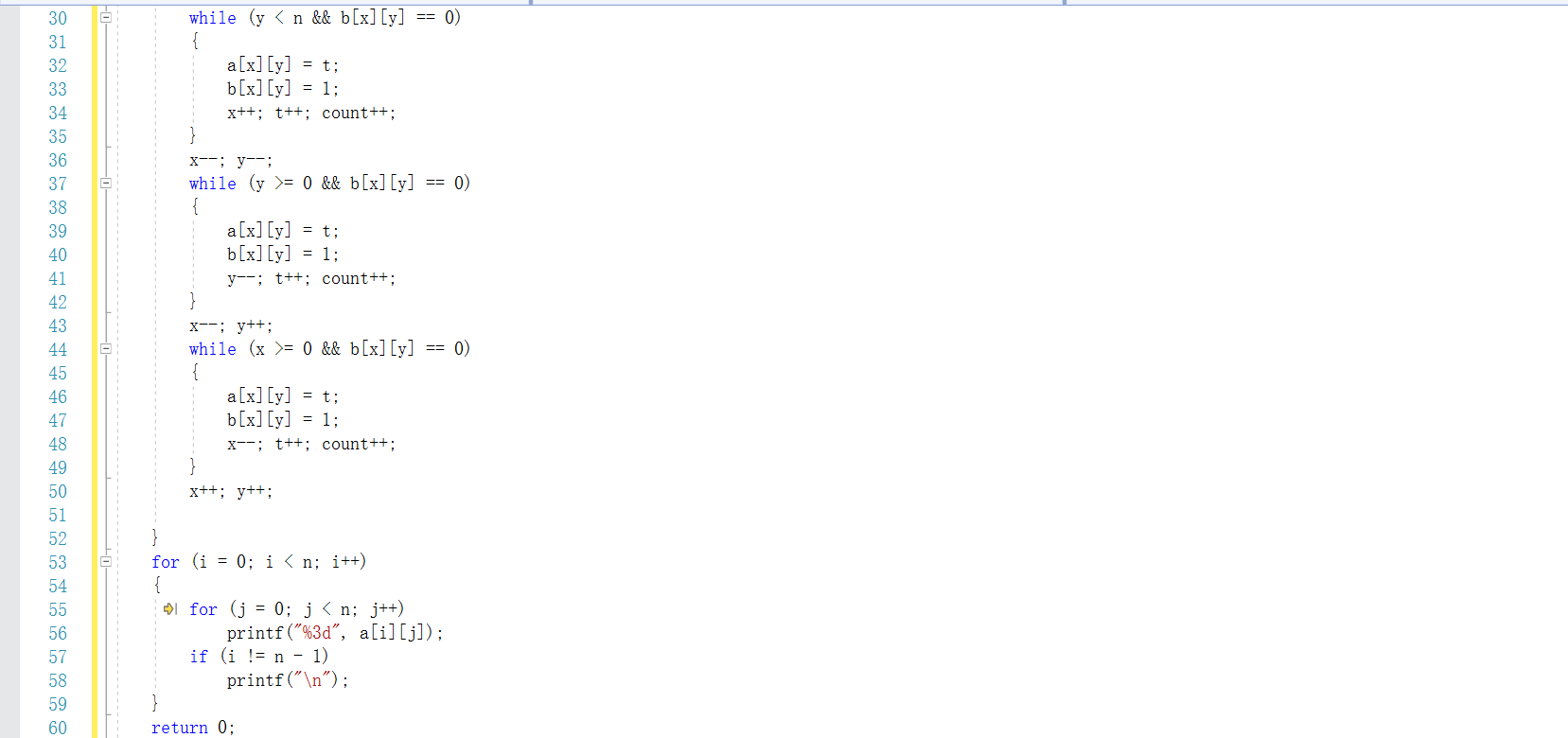## 2.1.3造测试数据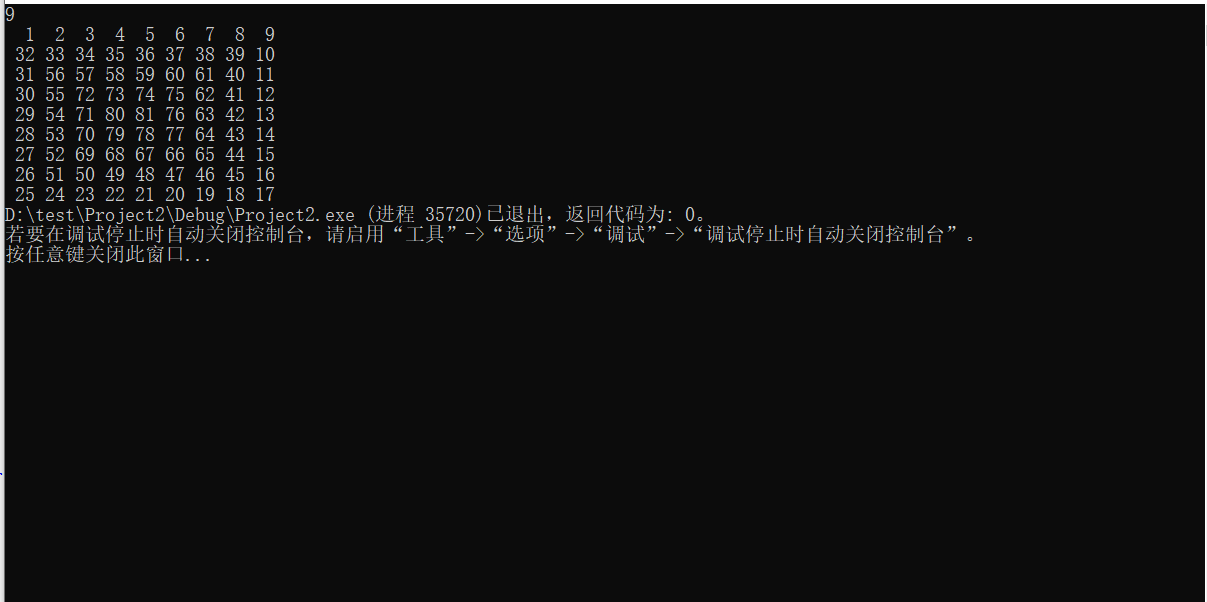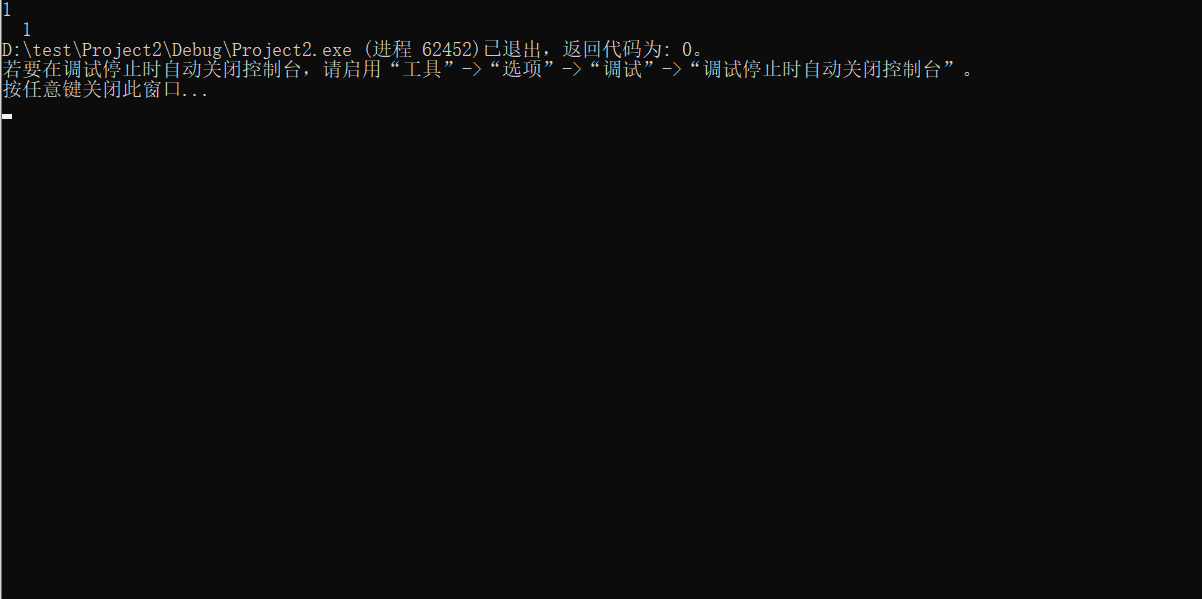## 2.1.4 PTA提交列表及说明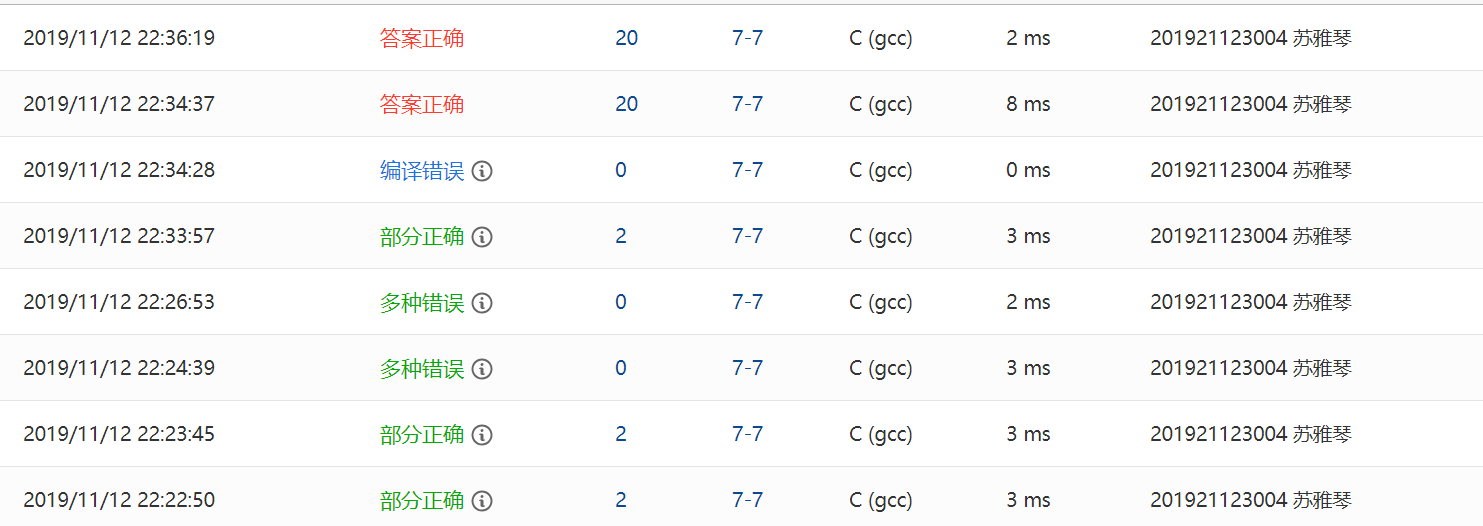Q1：部分错误：一开始，我其实对这道题没有思路，因为我纠结怎样让它一层一层的往里面输，所以后来借鉴了同学的代码，看了她的代码，知道是借助了另外一个数组。
Q2：部分错误：对于二维数组的初始化，一开始想着用b={0}这个方法简化一下程序，结果发现答案错误，原来二维数组的初始化和一维数组不一样，只能一个一个的去赋值。Q
Q3：一开始对b[i][j]的作业理解的不是很透彻



## 2.2 找鞍点

题目：一个矩阵元素的“鞍点”是指该位置上的元素值在该行上最大、在该列上最小。


### 2.2.1 伪代码

定义列最小变量minindex和行最大变量maxindex，循环变量i，j,k

for i=0 to i<n//寻找行最大值
maxindex=0
for j=0 to j<n-1
for k=0 to k<=n-1
if(a[i][minindex]<=a[i][k])
minindex=k;
end if
end for
end for
end for



### 2.2.2 代码截图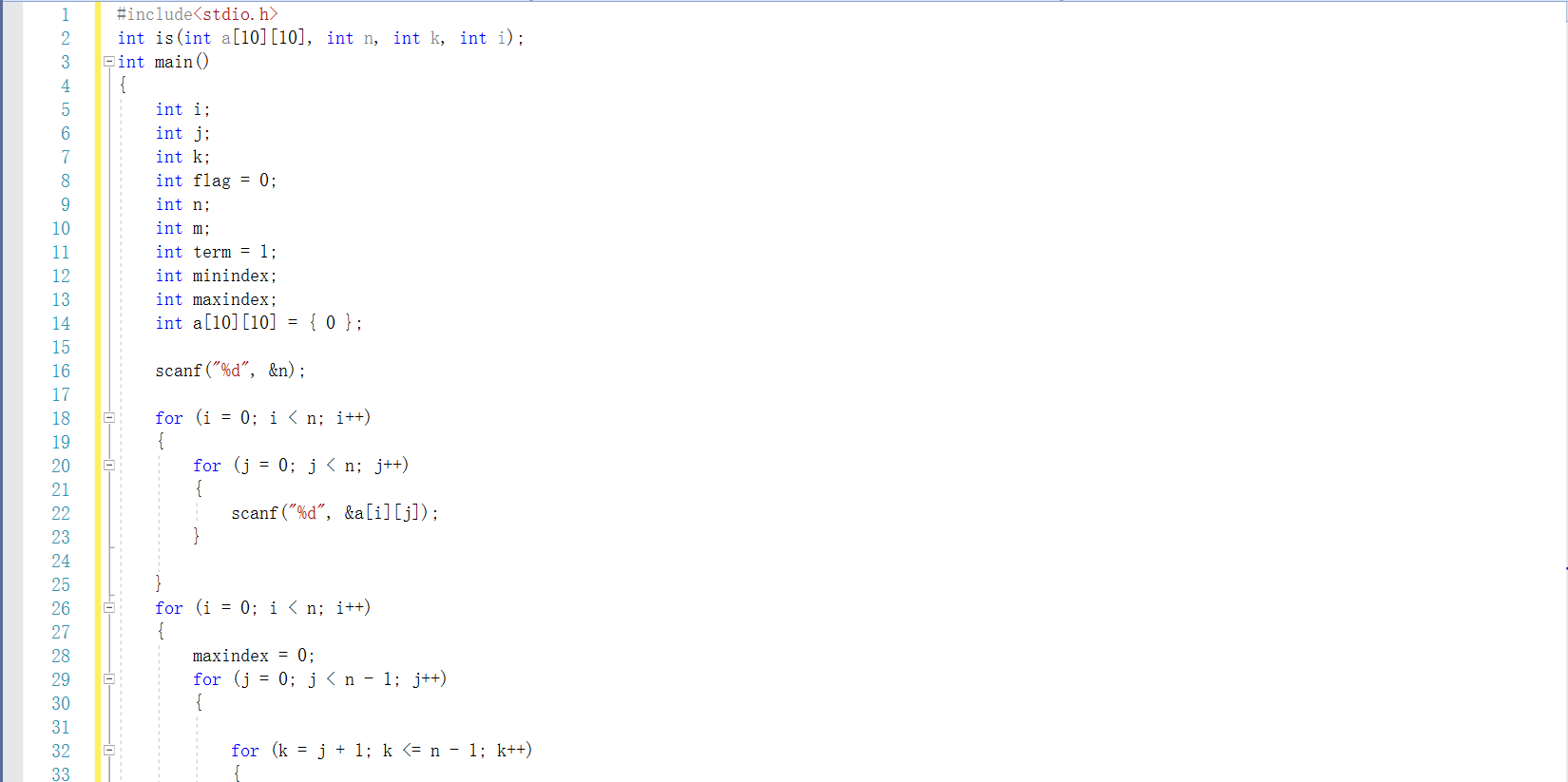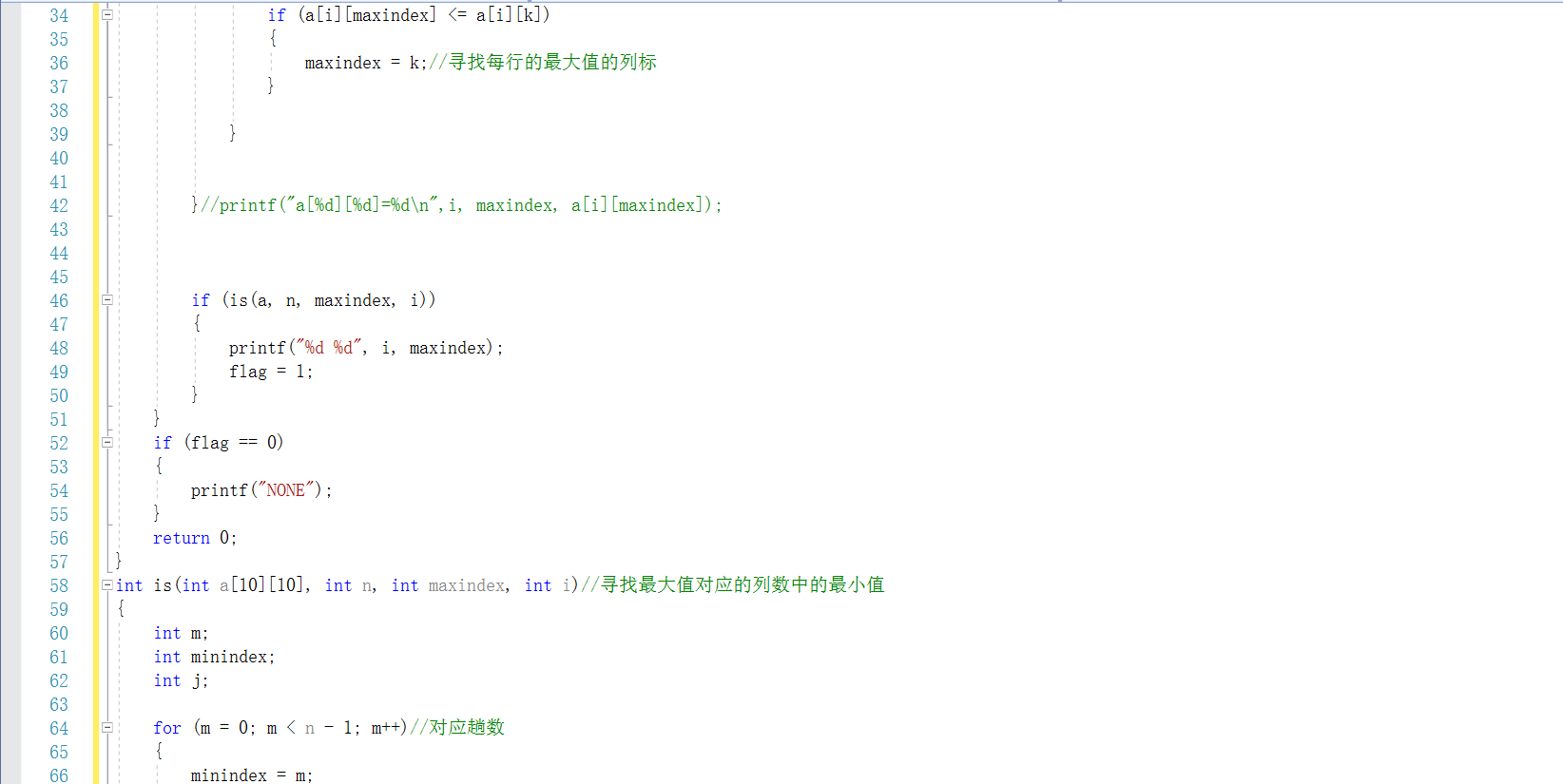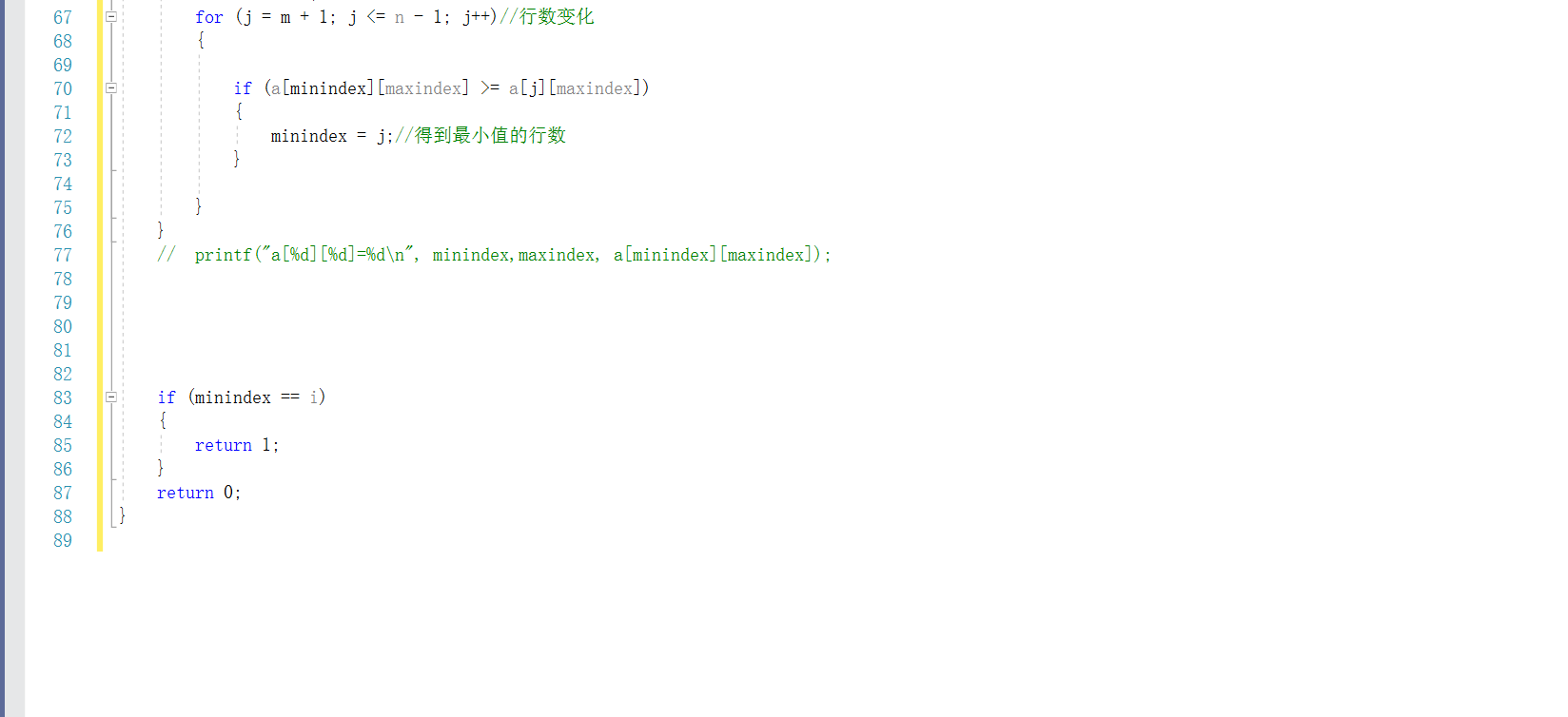sample1等价 答案正确
samole2等价 答案正确

### 2.2.4 PTA提交列表及说明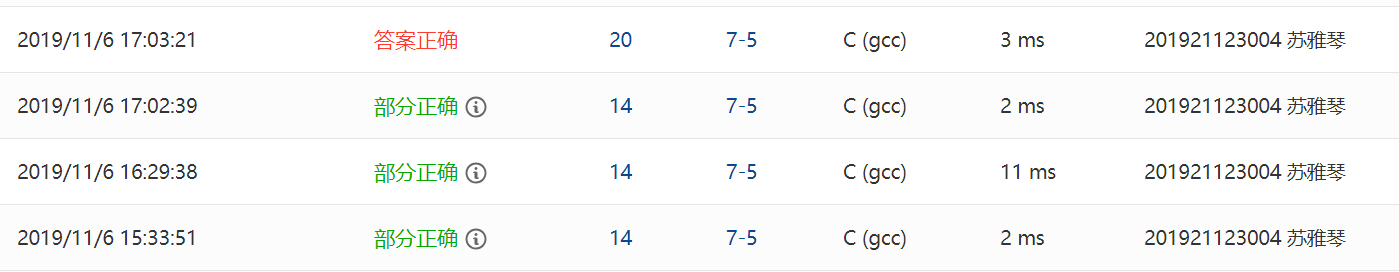Q1：部分正确：对于题目说至多存在一个鞍点没有理解清楚，所以发懵了好久。
Q2：部分正确：一开始循环条件没弄好，导致有鞍点的时候答案是NONE
Q3：部分正确：这题一开始其实不知道怎么结合函数一起做，所以都放在了main（）函数里，看起来就很乱，后来用函数把她们包装起来了。


## 2.3 阅览室

题目：天梯图书阅览室请你编写一个简单的图书借阅统计程序。当读者借书时，管理员输入书号并按下S键，程序开始计时；当读者还书时，管理员输入书号并按下E键，程序结束计时。书号为不超过1000的正整数。当管理员将0作为书号输入时，表示一天工作结束，你的程序应输出当天的读者借书次数和平均阅读时间。



### 2.3.1 伪代码

定义数组a[N],用来存放书号名，记录，和时间

for i=0 to i<n
while(1)
{
使用数组将数据存入数组
a[k][o]=num //存入书名
a[k]=op//存入存放记录
a[k]=hour*60+minute//存入时间
k++;
}

void AverageTime(int a[],int k);
{
for i=0 to i<k
{
if(存放记录a[i]是S)
{
for j=i+1 to j<=k
{
if(a[i]==a[j]&&a[j]=='S')
如果下面先遇到同一个书名的S，则break;
if(a[i]==a[j]&&a[j]=='E')
{
agv=agv+(a[j]-a[i]);//将时间累加
count++;//算次数
break;
}

}
}
}
if(count!=0)//以防万一除数是0
{
printf("%d %d.0f\n",count,agv/count);
}
else
printf("0 0\0");
}



### 2.3.2代码截图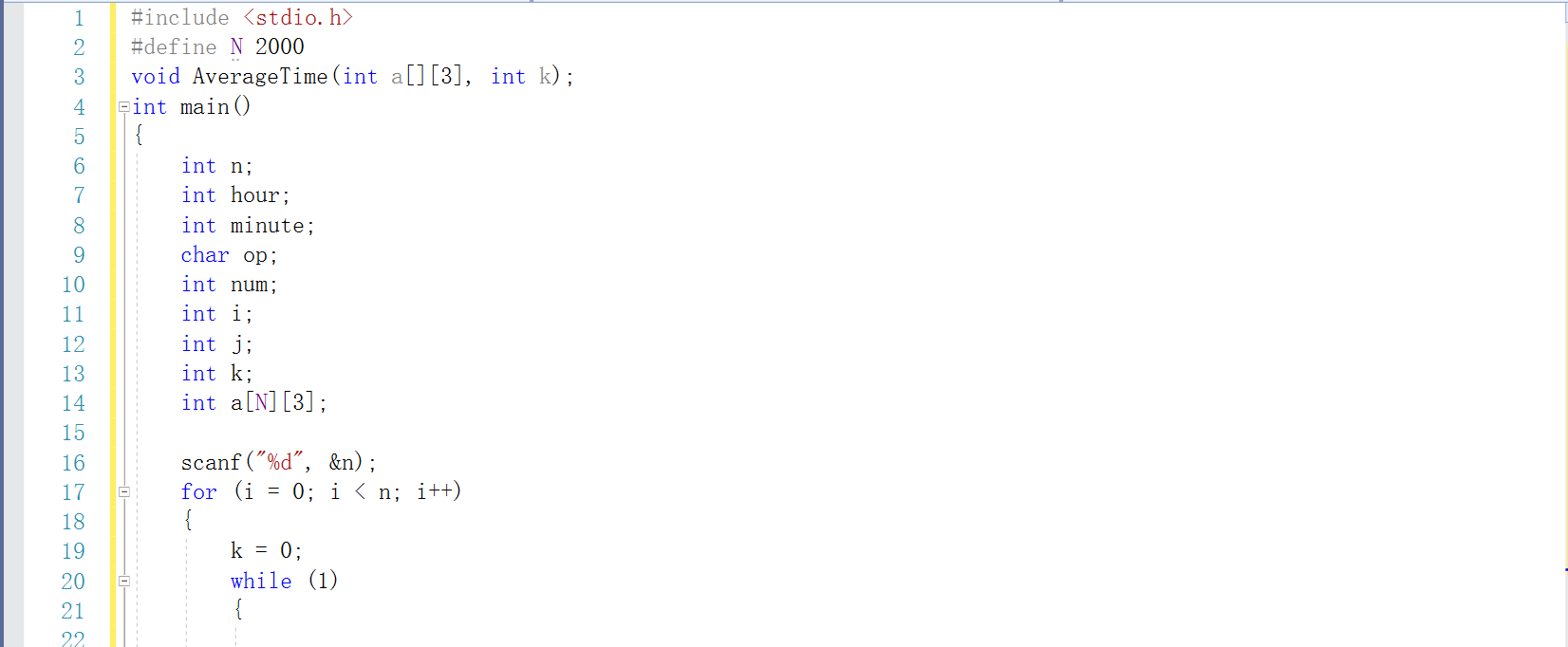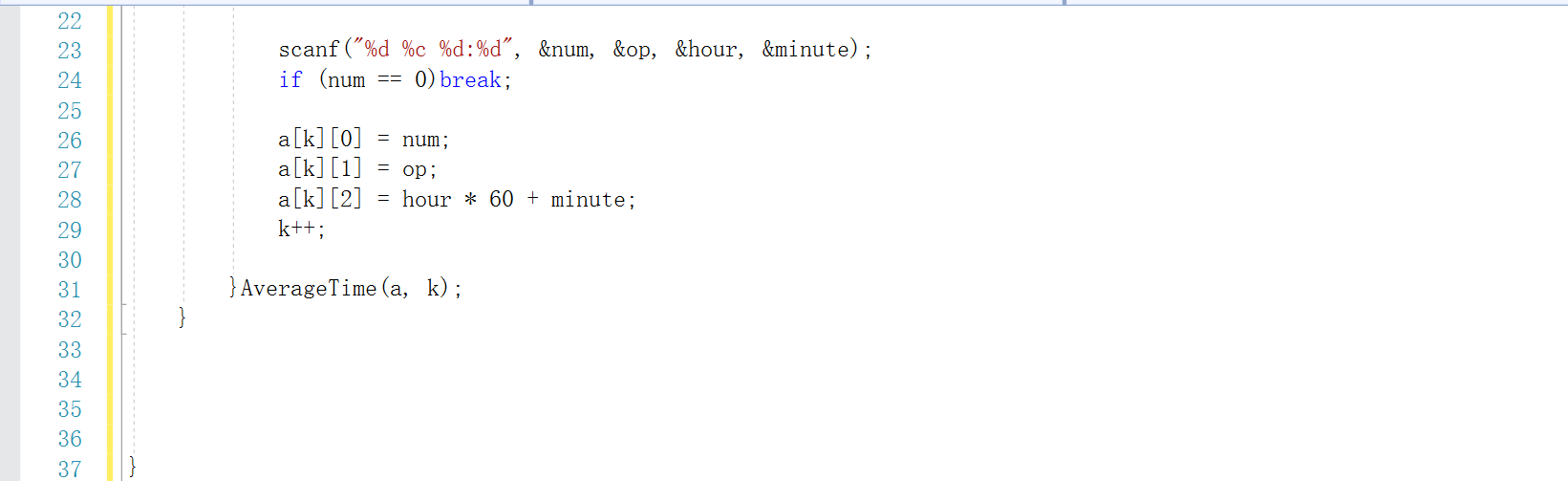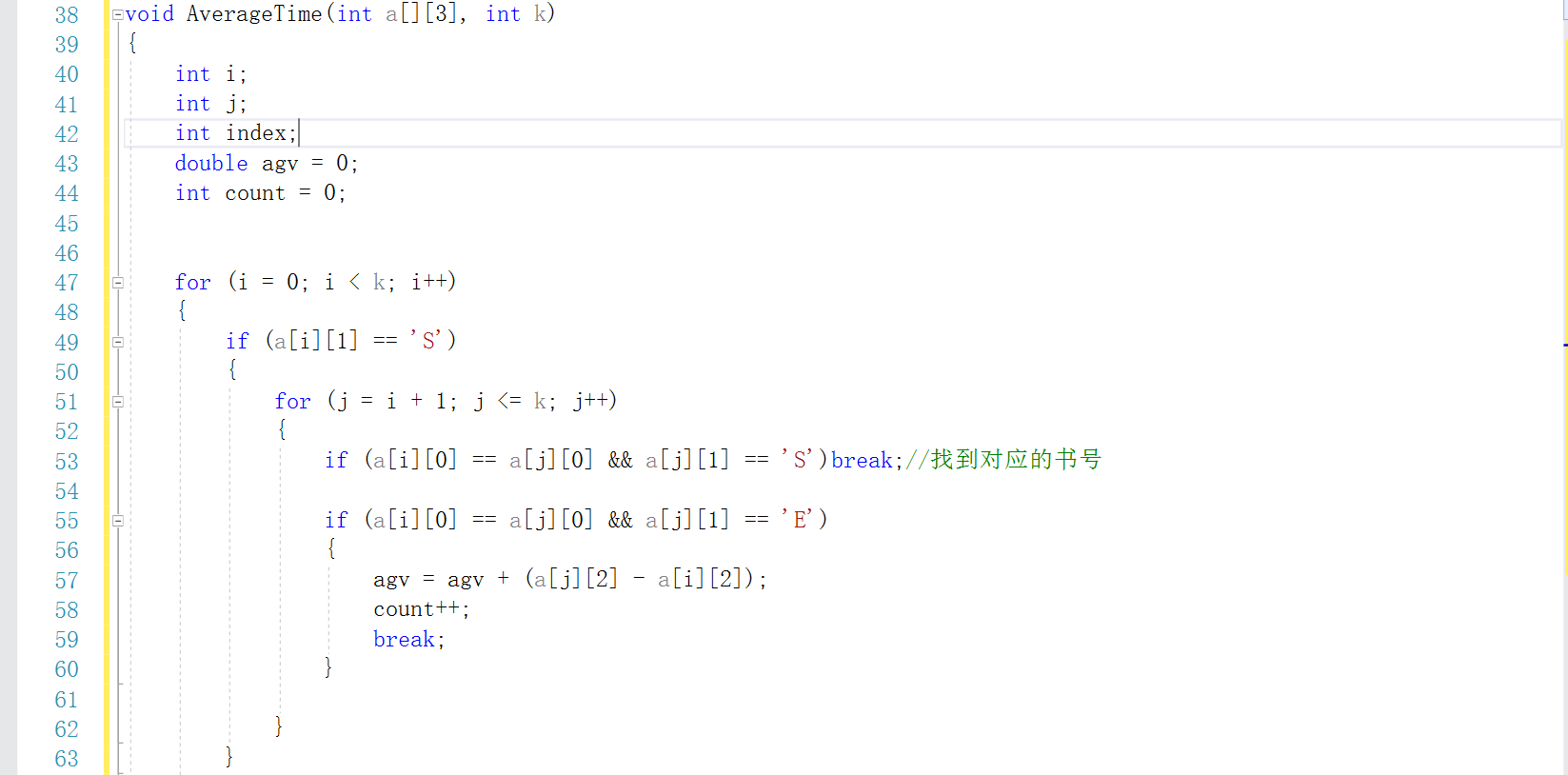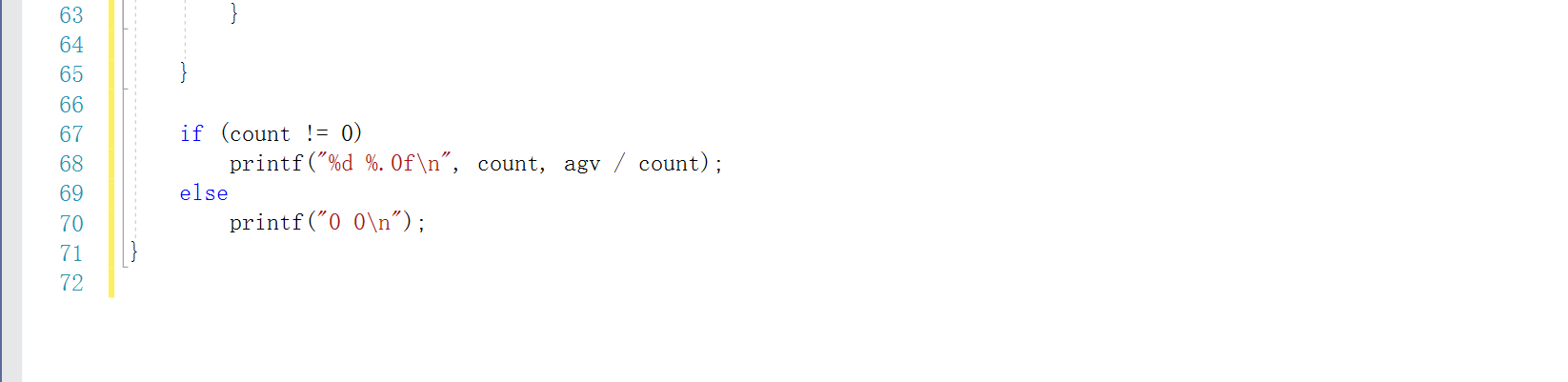### 2.3.3造测试数据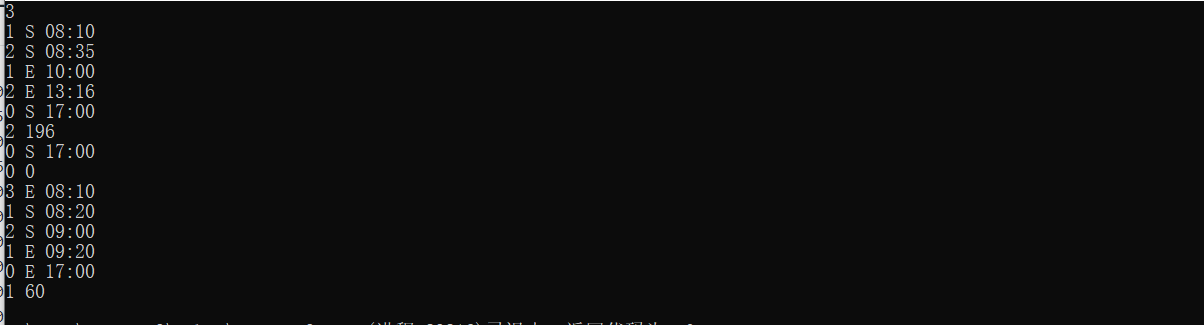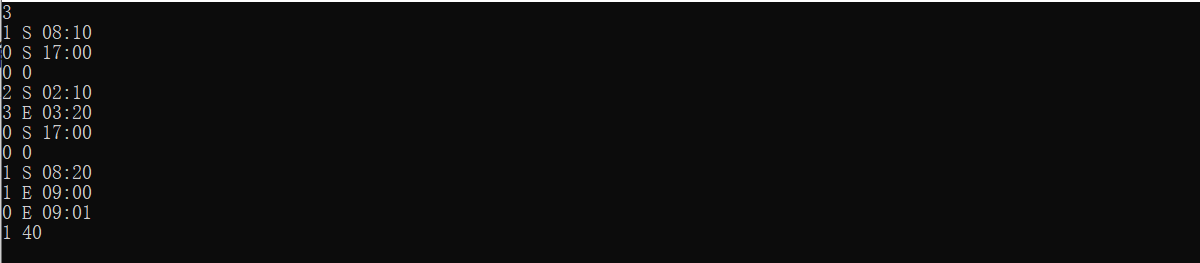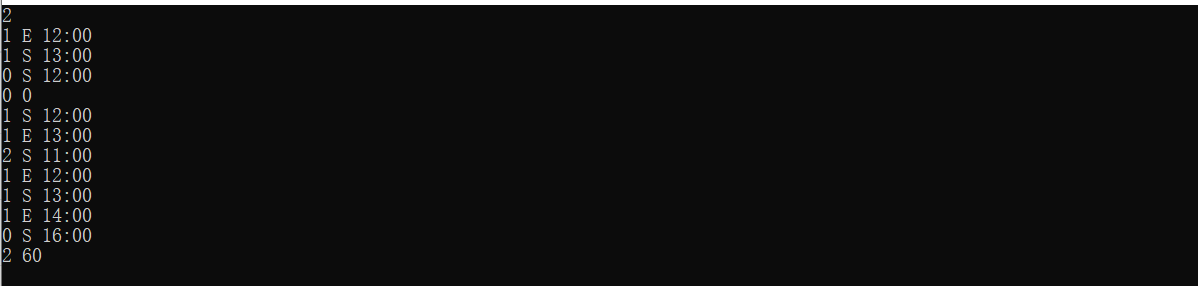sample等价 答案正确

E和S反掉 答案正确

### 2.3.4 PTA提交列表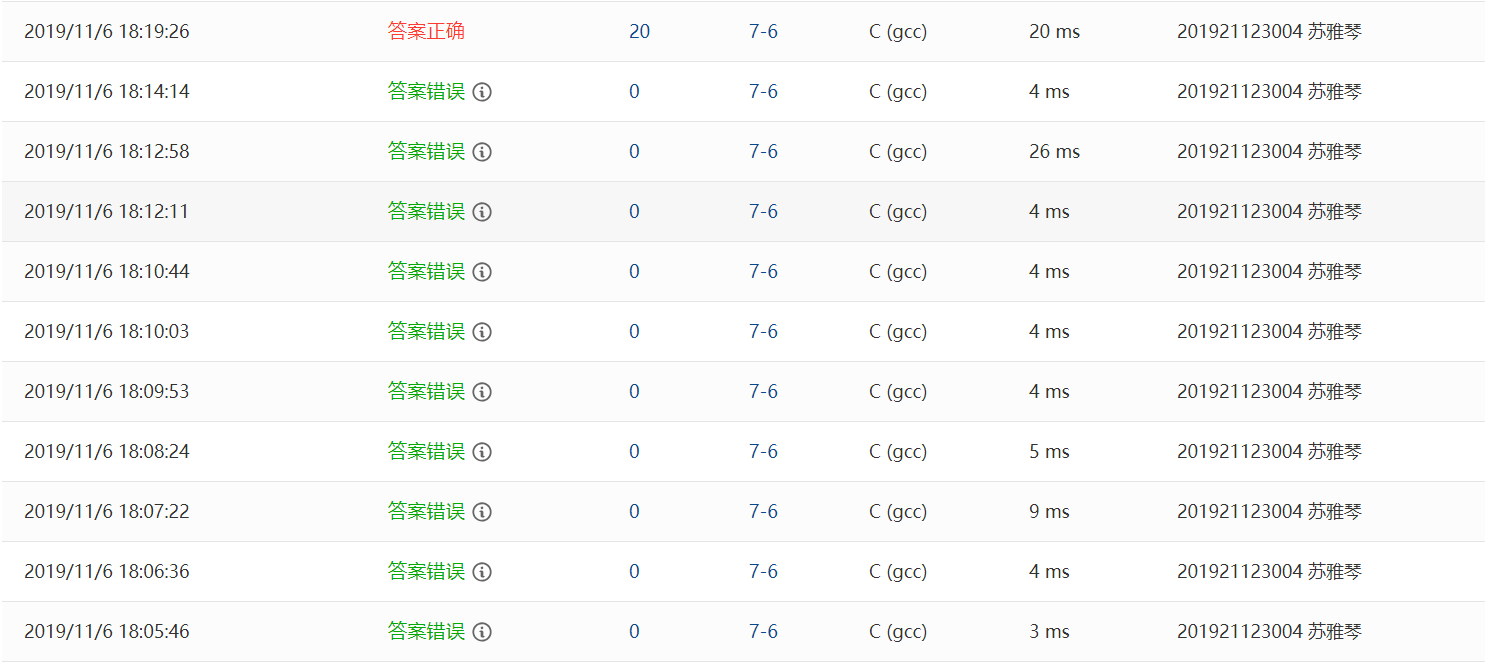Q1：答案错误：在主函数里循环变量忘记++了
Q2：答案错误：如果同一个书名出现两次借阅记录，应该按下面一次算
Q3：对题目理解不清，题目要求一天结束后就给出借阅次数和平均时间，但是我以为是一下子全部输出
Q4：答案错误：对于二维数组的利用没搞清楚
Q5：......


# 3.阅读代码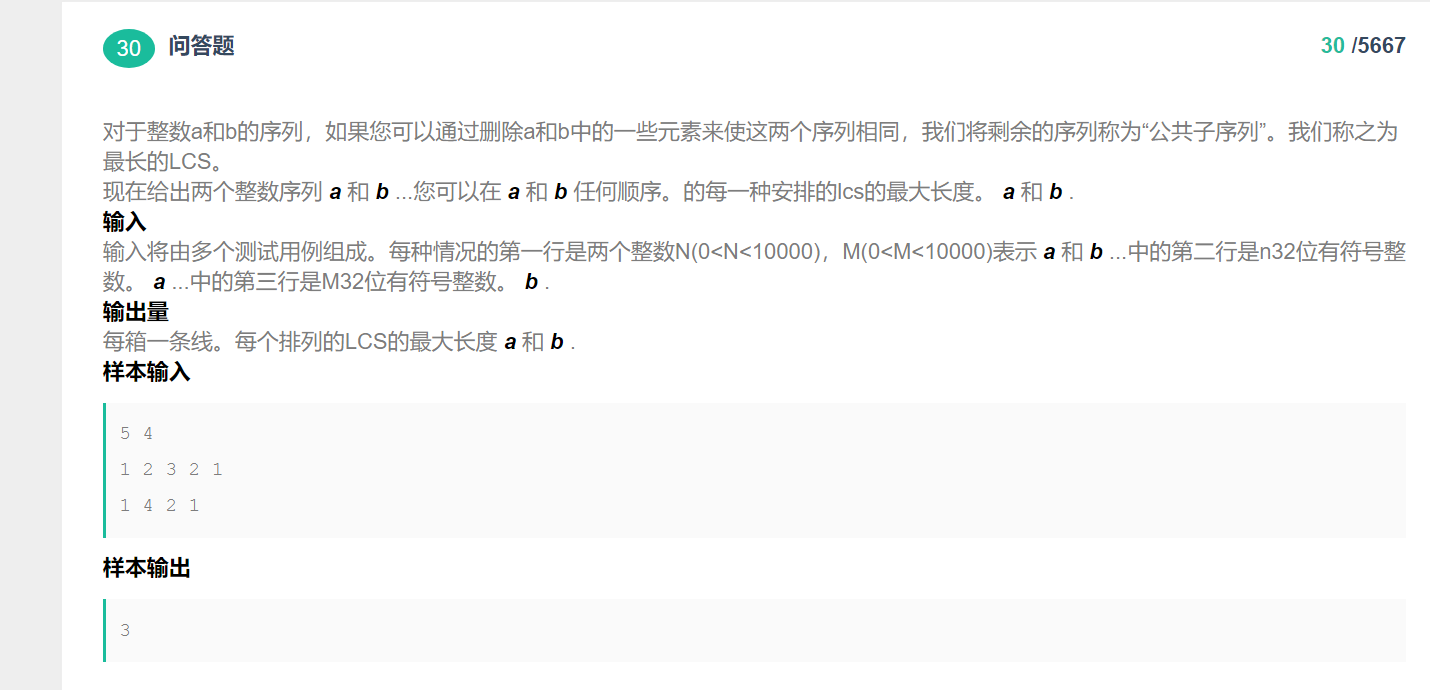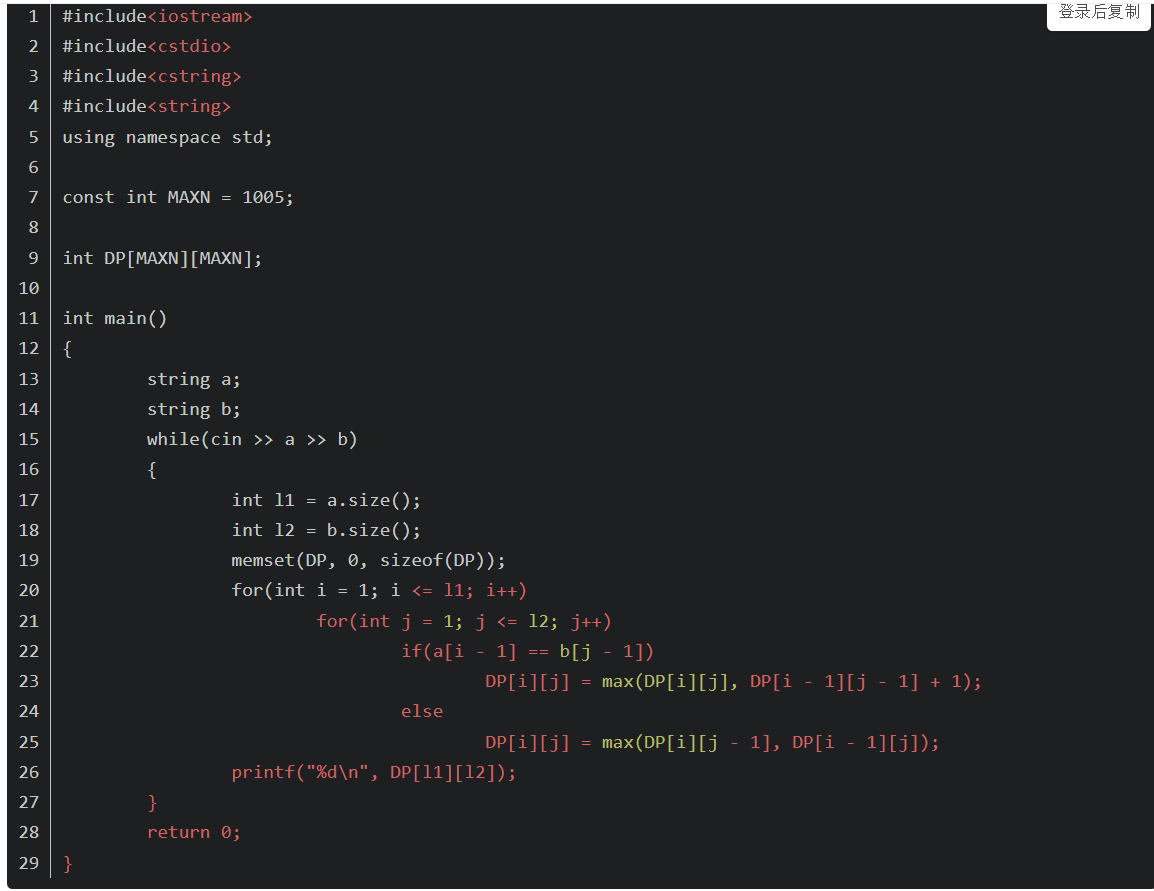### lcs（最长公共子序列）

• 以s1={1,3,4,5,6,7,7,8},s2={3,5,7,4,8,6,7,8,2}为例。 我们制作一个表格

•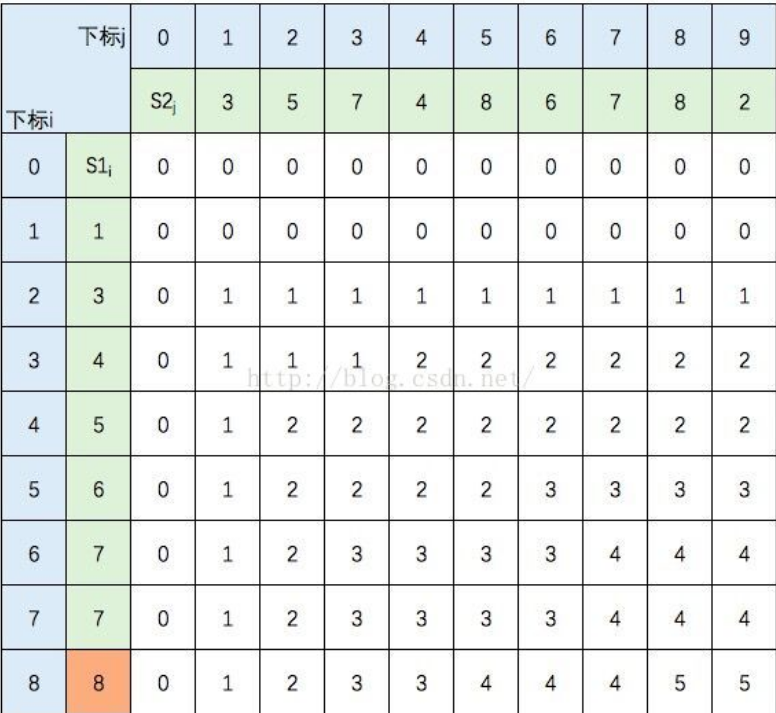• 根据下面规则填写表格，则 c[8,9] = S1 和 S2 的 LCS的长度，即为5。• 本题使用了动态规划，动态规划解决的问题多数有重叠子问题这个特点，为减少重复计算，对每一个子问题只解一次，将其不同阶段的不同状态保存在一个二维数组中，根据问题的可能性把问题划分成一步一步这样就可以通过递推或者递归来实现，选择最佳路径。
• 动态规划，是利用历史记录，来避免我们的重复计算。而这些历史记录，我们得需要一些变量来保存，一般是用一维数组或者二维数组来保存。
• 对于这个题目，我们要搞清楚数组元素的含义，然后找出数组元素之间的表达式，我觉得这就需要很强的思维能力，反正我做不来，到网上搜索了一下，才明白的一丢丢。 同时，这道题目还考察数学能力，我的天，我还是靠边站好了，要用到数学里的数学归纳法。不过我觉得这道题，其实让我学习更多的应该是如果用到二维数组，那么其中每个数与每个数之间的关系都是密不可分的，要深刻的去理解每个单元所包含的意思。
posted @ 2019-11-17 22:51  是啾咪呀。  阅读(857)  评论(0编辑  收藏  举报